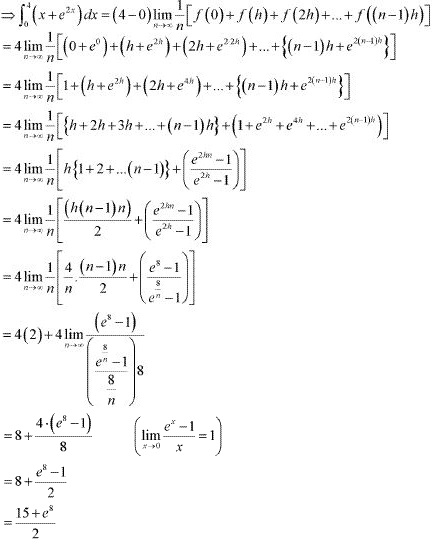# Class 12 Maths NCERT Solutions for Chapter 7 Integrals Exercise 7.8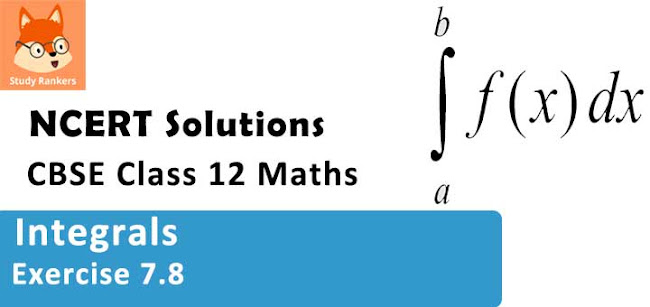### Integrals Exercise 7.8 Solutions

1. Evaluate the following definite integrals as limit of sums.

ab­ x dx

Solution

It is known that,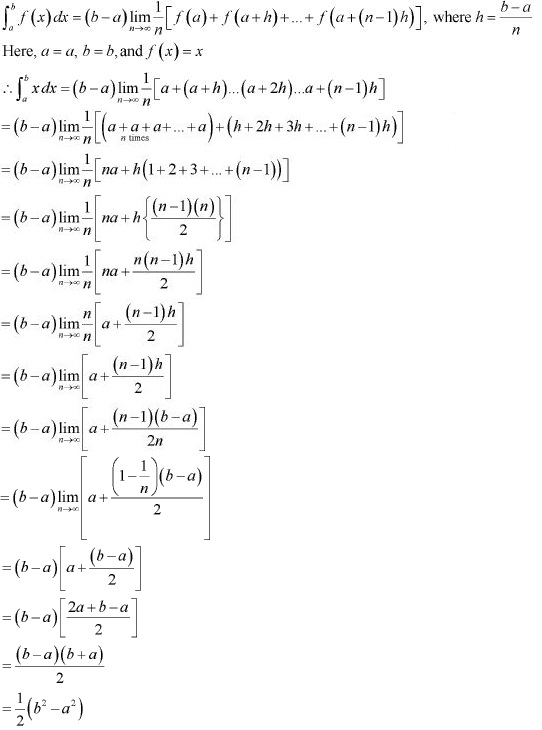2. Evaluate the following definite integrals as limit of sums.
ab­ (x + 1) dx

Solution

Let I = ab­ (x + 1) dx
It is known that,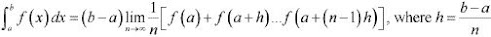Here, a = 0, b = 5, and f(x) = (x + 1)
⇒ h = (5 - 0)/n = 5/n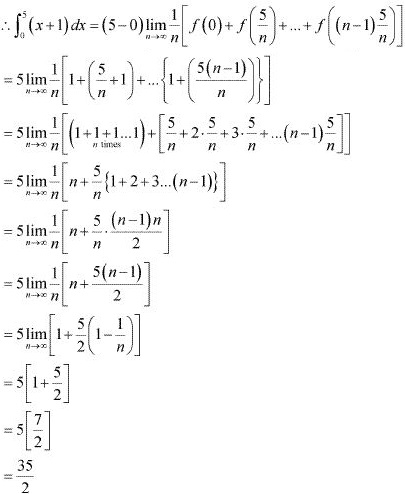3. 23­ x2 dx

Solution

It is known that,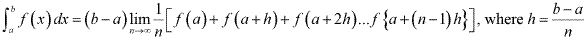Here, a = 2, b = 3, and f(x) = x2
⇒ h = (3 - 2)/n = 1/n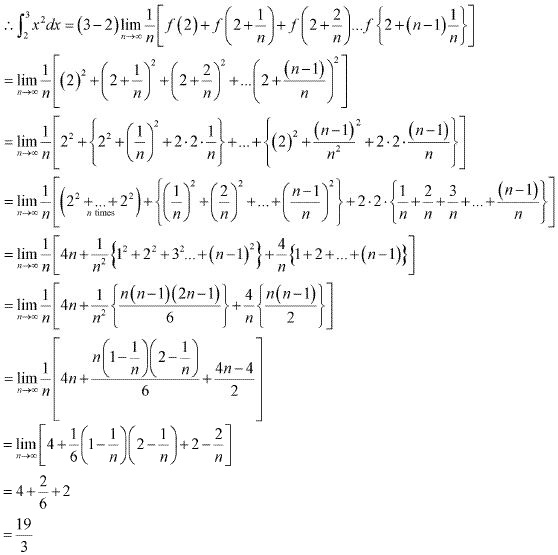4. 23­ (x2 - x) dx

Solution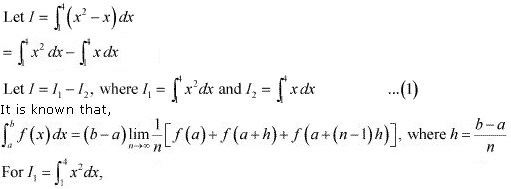a = 1, b = 4, and f(x) = x2
∴ h = (4 - 1)/n = 3/n= 3[1 + 3 + 3]
= 3(7)
I1 = 21 ...(2)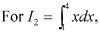a = 1, b = 4, and f(x) = x
⇒ h = (4 -1)/n = 3/n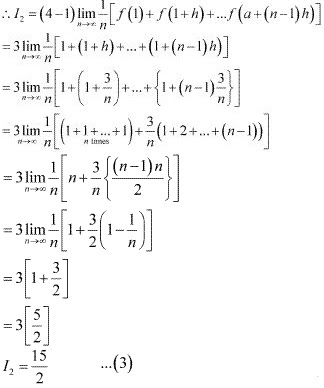From equations (2) and (3), we obtain
I2  = 15/2 ...(3)
From equations (2) and (3), we obtain
I = I1 + I2 = 21 - 15/2 = 27/2

5. Evaluate the following definite integrals as limit of sums  -11­ ex dx
Solution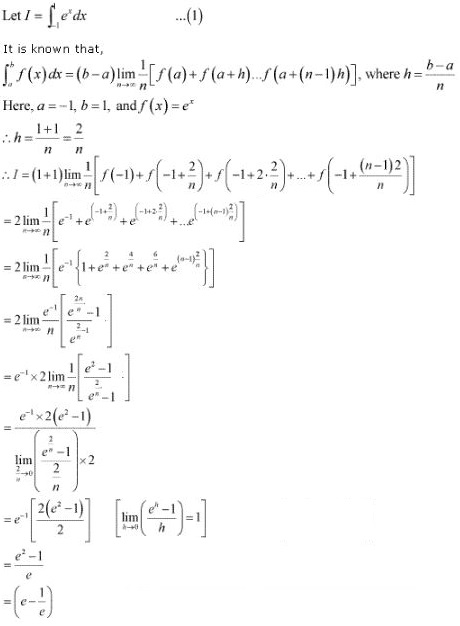6. Evaluate the following definite integrals as limit of sums 04­ (x + e2x ) dx
Solution
It is known that ,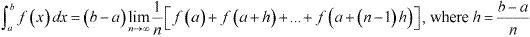Here, a = 0, b = 4, and f(x) = x + e2x
∴h = (4 - 0)/n = 4/n